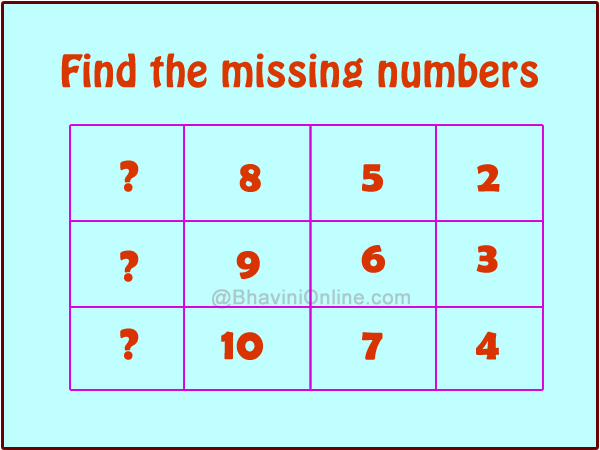# Number Series Riddle: Find the Missing Numbers in the Box

Let\’s have fun with numbers;

In the given box you will see the numbers are connected in some way.

Find the connection between the numbers and then find the missing numbers;In text the box can be displayed as follows;

 ? 8 5 2 ? 9 6 3 ? 10 7 4

If you see the table properly, you will see that it is easier to solve the riddle when you solve it right to left.

You have to keep adding 3 to the numbers on the right to get the numbers on the left.

So in first row;

2 + 3 = 5

5 + 3 = 8

So we can find ? by;

8 + 3 = 11

Similarly we can solve column 2 and 3 to get the next number;

9 + 3 = 12 &

10 + 3 = 13

So Answer is 11, 12 & 13

 11 8 5 2 12 9 6 3 13 10 7 4